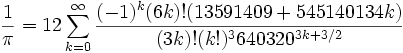Thanks to metacircular for pointing out that (floor (/ x y)) can be written as (floor x y) while avoiding
the intermediate rational.

```(defun machin-pi (digits)
"Calculates PI digits using fixed point arithmetic and Machin's formula with double recursion"
(labels
((arccot-minus (xsq n xpower)
(let ((term (floor xpower n)))
(if (= term 0)
0
(- (arccot-plus xsq (+ n 2) (floor xpower xsq))
term))))
(arccot-plus (xsq n xpower)
(let ((term (floor (/ xpower n))))
(if (= term 0)
0
(+ (arccot-minus xsq (+ n 2) (floor xpower xsq))
term))))
(arccot (x unity)
(let ((xpower (floor (/ unity x))))
(arccot-plus (* x x) 1 xpower))))
(let* ((unity (expt 10 (+ digits 10)))
(thispi (* 4 (- (* 4 (arccot 5 unity)) (arccot 239 unity)))))
(floor thispi (expt 10 10)))))
```

The first 10000 digits again.

```* (time (machin-pi 10000))
Evaluation took:
0.662 seconds of real time
0.634038 seconds of total run time (0.495454 user, 0.138584 system)
[ Run times consist of 0.233 seconds GC time, and 0.402 seconds non-GC time. ]
95.77% CPU
1,491,387,858 processor cycles
109,530,592 bytes consed
31415926535897932384626433832795028841971693993751058209749445923078164062862089 ...
```

Algorithmic optimizations would take us much further. For example the Gauss–Legendre or Salamin–Brent formula.
Then there is the fastest known(at the turn of the millenium), Chudnovsky’s formula :